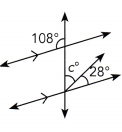# Math in Focus Grade 8 Chapter 9 Answer Key Congruence and Similarity

This handy Math in Focus Grade 8 Workbook Answer Key Chapter 9 Congruence and Similarity detailed solutions for the textbook questions.

## Math in Focus Grade 8 Course 3 B Chapter 9 Answer Key Congruence and Similarity

### Math in Focus Grade 8 Chapter 9 Quick Check Answer Key

Complete.

Question 1.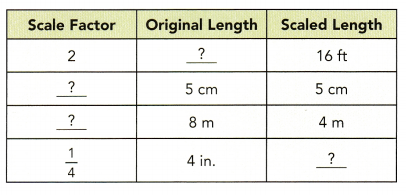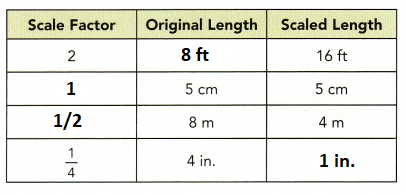Explanation:
1. Scale factor: 2
2 × 8ft = 16 ft
2. Original length = 5
Scaled length = 5
Scale factor = 5/5 = 1
3. Original length = 8 m
Scaled length = 4 m
Scale factor = scale/actual = 4/8 = 1/2
4. Scale factor = 1/4
Original length = 4 inches
We know that
scale factor = scale/actual
1/4 = scale length/4
scaled length = 4/4
scaled length = 1 in.

Solve.

Question 2.
Shawn built a model of a ship whose length was 4,500 inches. His model was 15 inches long. Find the scale factor.
Let the scale factor be x
Shawn built a model of a ship whose length was 4,500 inches.
His model was 15 inches long.
x × 15 = 4500
x = 4500/15
x = 300 inches

Question 3.
A line segment is 4 centimeters long. When projected on a screen, the line segment is 20 centimeters long. Find the scale factor.
Let the scale factor be x
scale factor = scale/actual
Actual length = 4 cm
scaled length = 20 cm
scale factor = 20 cm/4 cm
scale factor = 5 cm
Thus the scale factor is 5 cm.

Question 4.
A model plane is built with a scale factor of $$\frac{1}{180}$$. The actual length of the plane is 210 feet (2,520 inches). Find the length of the model.
Given,
A model plane is built with a scale factor of $$\frac{1}{180}$$.
The actual length of the plane is 210 feet (2,520 inches).
Scale/Actual = 1/180
1/180 = l/210 feet
210/180 = length
Length = 210/180
Length = 21/18
Length = 7/6
Length = 1.16 feet

Question 5.
The scale of a map is 2 inches : 3 kilometers. The length of a road on the map is 3 inches. Find the actual length of the road.
Given,
The scale of a map is 2 inches : 3 kilometers.
The length of a road on the map is 3 inches
Let the actual length of the road be x.
2 in./ 3 km = 3 in / x km
x = (3 × 3)/2
x = 9/2 = 4.5 km
Thus the actual length of the road is 4.5 kilometers.

Question 6.
The diagram shows a plot of land ABCD drawn on a map.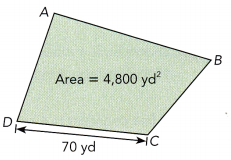a) On the map, $$\overline{C D}$$ is 1$$\frac{3}{4}$$ inches. Find the scale of the map.

b) Find the area of ABCD on the map.

The measures of two interior angles are given for each triangle. Find the measure of the third interior angle.

Question 7.
△ABC: 20°, 80°
Given
△ABC: 20°, 80°
The sum of interior angles of the triangle = 180°
∠a + ∠b + ∠c = 180°
20°+ 80° + ∠c = 180°
100° + ∠c = 180°
∠c = 180° – 100°
∠c = 80°
Thus the measure of the third interior angle is 80°

Question 8.
△KLM: 37°, 76°
Given,
△KLM: 37°, 76°
The sum of interior angles of the triangle = 180°
∠k + ∠l + ∠m = 180°
37°+ 76° + ∠m = 180°
113° + ∠m = 180°
∠m = 180° – 113°
∠m = 67°
Thus the measure of the third interior angle is 67°

Question 9.
△PQR: 15°, 103°
Given,
△PQR: 15°, 103°
The sum of interior angles of the triangle = 180°
∠p + ∠q + ∠r = 180°
15°+ 103° + ∠r = 180°
∠r = 180° – 118°
∠r = 62°

Find the unknown angle measure.

Question 10.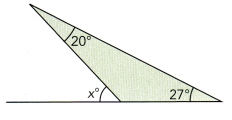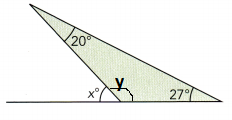Let the unknown angle be y
The sum of three angles = 180°
20° + 27° + y = 180°
47° + y = 180°
y = 180° – 47°
y = 133°
x° + y° + 27° = 180°
x° + 133°  + 27° = 180°
x° = 180° – 133° – 27°
x° = 20°
Thus the unknown angle is 20°

Question 11.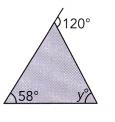y° + y° = 120°
2y° = 120°
y = 120°/2
y =60°

Solve for each variable.

Question 12.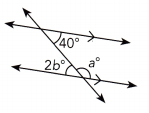a° + 2b° = 180
b° = 40° (alternate angles)
2b° = 80°
a° + 2b° = 180
a° + 80° = 180°
a° = 180° – 80°
a° = 100°

Question 13.